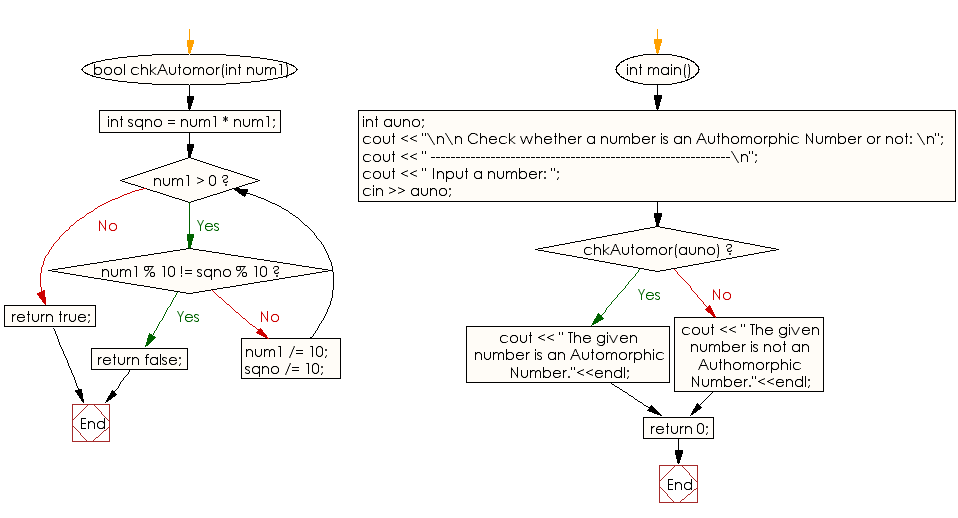﻿ C++ Exercises: Check if a number is Authomorphic or not - w3resource

# C++ Exercises: Check if a number is Authomorphic or not

## C++ Numbers: Exercise-24 with Solution

Write a program in C++ to check if a number is Authomorphic or not.

Sample Solution:

C++ Code :

``````#include<iostream>
using namespace std;
bool chkAutomor(int num1)
{
int sqno = num1 * num1;
while (num1 > 0)
{
if (num1 % 10 != sqno % 10)
return false;
num1 /= 10;
sqno /= 10;
}
return true;
}
int main()
{
int auno;
cout << "\n\n Check whether a number is an Authomorphic Number or not: \n";
cout << " ------------------------------------------------------------\n";
cout << " Input a number: ";
cin >> auno;

if( chkAutomor(auno))
cout << " The given number is an Automorphic Number."<<endl;
else
cout << " The given number is not an Authomorphic Number."<<endl;
return 0;
}
``````

Sample Output:

```Check whether a number is an Authomorphic Number or not:
------------------------------------------------------------
Input a number: 25
The given number is an Automorphic Number.
```

Flowchart:C++ Code Editor: